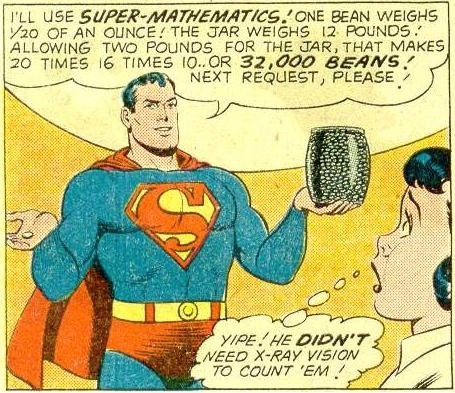## Poll

 I love math!17 votes (50%) Math is great.13 votes (38.23%) My religion is mathology.5 votes (14.7%) Women didn't speak to me until I was 30.2 votes (5.88%) Total eclipse reminder -- 04/08/202411 votes (32.35%) I steal cutlery from restaurants.3 votes (8.82%) I should just say what's on my mind.6 votes (17.64%) Who makes up these awful names for pandas?5 votes (14.7%) I like to touch my face.10 votes (29.41%) Pork chops and apple sauce.8 votes (23.52%)

34 members have voted

WizardJoined: Oct 14, 2009
• Posts: 23444
June 12th, 2021 at 9:42:46 PM permalink
A circle of radius 1 is inscribed in an equilateral triangle. What is the area of the triangle?
It's not whether you win or lose; it's whether or not you had a good bet.
Ace2Joined: Oct 2, 2017
• Posts: 1070
June 12th, 2021 at 10:21:15 PM permalink
Quote: Wizard

A circle of radius 1 is inscribed in an equilateral triangle. What is the area of the triangle?

3 * 3^.5 or about 5.2
It�s all about making that GTA
gordonm888Joined: Feb 18, 2015
• Posts: 3277
June 13th, 2021 at 12:02:48 AM permalink
What is wrong with this picture below?So many better men, a few of them friends, are dead. And a thousand thousand slimy things live on, and so do I.
acesideJoined: May 14, 2021
• Posts: 65
June 13th, 2021 at 3:52:18 AM permalink
Quote: gordonm888

What is wrong with this picture below?I can solve this one. It�s 32,00 beans.
acesideJoined: May 14, 2021
• Posts: 65
June 13th, 2021 at 3:56:05 AM permalink
Quote: Ace2

Quote: ThatDonGuy

47,070,163,786,203,595,393,879,102,723 / 1,237,940,039,285,380,274,899,124,224, which is about 38.

I disagree. My answer is significantly higher than yours.

I did a basic simulation in excel and there is only about a 1 in 3,000 chance of success with that many draws

How do you do simulations in excel? I�d like to learn this skill for my simulating some blackjack side bets.
ThatDonGuyJoined: Jun 22, 2011
• Posts: 4962
June 13th, 2021 at 6:46:54 AM permalink
Quote: Wizard

A circle of radius 1 is inscribed in an equilateral triangle. What is the area of the triangle?

Let A, B, C be the vertices of the triangle, O the center of the circle,
and D, E, F be the points where the circle is tangent to sides AB, AC, and BC, respectively.
OD = OE = OF = the radius of the circle, which is 1.
AEO and AFO are both right angles.
EO = FO = 1 and AO = AO, so right triangles AEO and AFO are congruent (Hypotenuse-Leg), which means angle OAE = angle OAF = 1/2 of 60 degrees = 30 degrees, and AOE is a 30-60-90 triangle. with the side opposite the 30-degree angle having length 1, so AE has length sqrt(3).
Using the same methods on COD and COE, CE also has length sqrt(3), so the length of AC = 2 sqrt(3).
The altitude of the triangle from B to AC divides it into two 30-60-90 triangles with the side opposite the 30-degree angle = sqrt(3), so the altitude = 3, and the area = 1/2 x 2 sqrt(3) x 3 = 3 sqrt(3).

charliepatrickJoined: Jun 17, 2011
• Posts: 2444
June 13th, 2021 at 7:30:18 AM permalink
Quote: ThatDonGuy

"Not a Wild Guess"...

I agree but here's a slightly different method of proof.
Assuming AB is the lower side of the triangle and D is the tangent, then consider triangle OAD. The height is 1, as it's the radius. One angle (BAO) is half BAC, so is 30 degrees. Thus the hypotenuse is 2, and the base is SQRT(4-1)=SQRT(3). So the area of that triangle (1/2 b h) is SQRT(3)/2. There are six of these triangles (which make up the ABC triangle) so the total area is 3 SQRT(3).
Ace2Joined: Oct 2, 2017
• Posts: 1070
June 13th, 2021 at 10:19:17 AM permalink
I looked up a equilateral triangle on wikipedia where it says:

radius of an inscribed circle = 3^.5 * (side) / 6 = 1
area of triangle = 3^.5 * (side)^2 / 4

Very easy to solve with that
It�s all about making that GTA
WizardJoined: Oct 14, 2009
• Posts: 23444
June 13th, 2021 at 4:29:42 PM permalink
Quote: Ace2

3 * 3^.5 or about 5.2

I agree!
It's not whether you win or lose; it's whether or not you had a good bet.
ThatDonGuyJoined: Jun 22, 2011